Like   Tweet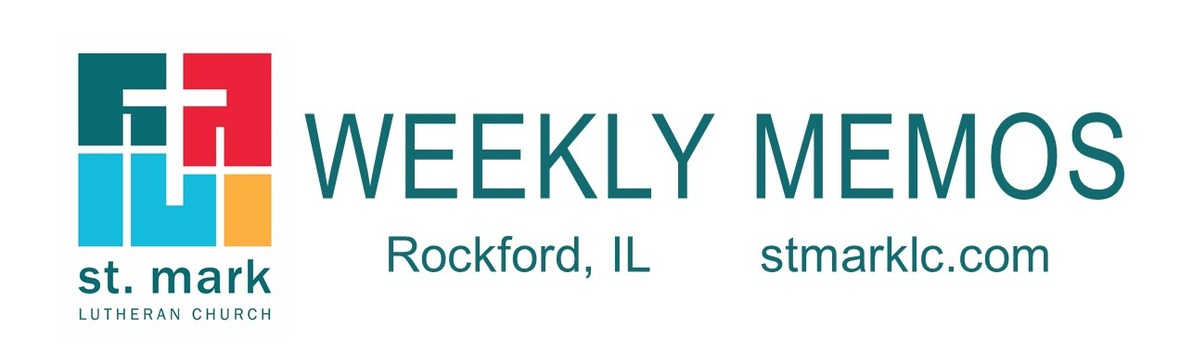## This Week at St. Mark December 16-23, 2018

 table div table+table+table div table{width:100%;padding:0}table div table+table+table div table img{width:96.23%;padding:0;float:none}table div table+table+table div table td{width:100%;padding:0 1.88% 18px}/* styles */## Sunday, December 16 Third Sunday of Advent

8:00 A.M. Worship
9:30 A.M. Worship
9:30 A.M. Sunday School
11:00 A.M. Worship

## Monday, December 17

6:30 P.M. Emotions Anonymous
6:30 P.M. Blue Lights
6:30 P.M. Ukulele Club

## Tuesday, December 18

10:00 A.M. Staff Meeting
11:00 A.M. St. Mark Duplicate Bridge

## Wednesday, December 19

9:00 A.M. Men's Bible Study
9:00 A.M. Women's Bible Study
5:30 P.M. Good News Bearers
6:15 P.M. Bible Study
6:30 P.M. Confirmation
7:00 P.M. Voices of St. Mark

## Thursday, December 20

7:30 A.M. Panera Book Study
9:00 A.M. Cherry Valley Bridge Group
6:30 P.M. Emotions Anonymous
6:30 P.M. Diakonia
7:00 P.M. Praise Band Rehearses

## Sunday, December 23 4th Sunday of Advent

8:00 A.M. Worship
9:30 A.M. Worship
11:00 A.M. Worship

 table div table+table+table+table+table+table div table{width:100%;padding:0}table div table+table+table+table+table+table div table img{width:96.23%;padding:0;float:none}table div table+table+table+table+table+table div table td{width:100%;padding:0 1.88% 18px}/* styles */## News and Events

 table div table+table+table+table+table+table+table+table div table{width:100%;padding:0}table div table+table+table+table+table+table+table+table div table img{width:96.23%;padding:0;float:none}table div table+table+table+table+table+table+table+table div table td{width:100%;padding:0 1.88% 18px}/* styles */The Congregation Council is hosting a farewell reception for Karen Kammer this Sunday following the 8:00 and 9:30 services. We hope you will all join us in making this a special day for Karen. Thank you, Karen, for 21 years of faithful service to St. Mark. Enjoy your retirement.

 table div table+table+table+table+table+table+table+table+table+table div table{width:100%;padding:0}table div table+table+table+table+table+table+table+table+table+table div table img{width:96.23%;padding:0;float:none}table div table+table+table+table+table+table+table+table+table+table div table td{width:100%;padding:0 1.88% 18px}/* styles */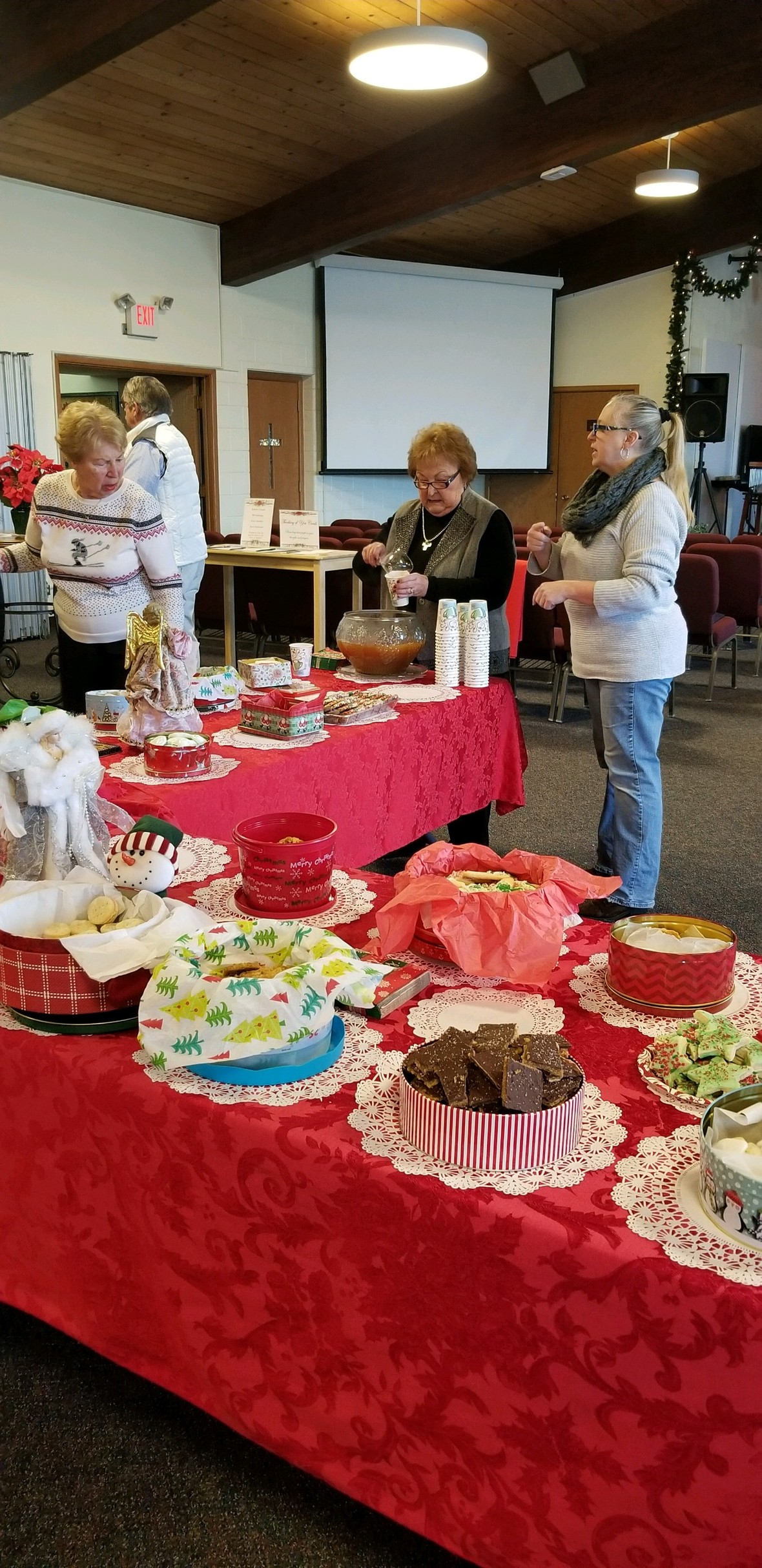Thank You
The Primetimers would like to take the opportunity to thank all the bakers who brought in their tins of homemade cookies. They were not only decorated professionally and baked with love, but they also tasted oh so good!. Thank you one and all. A special thank you to Karla & Les, who prepared the warm Wassail. You know who they are, you know, the two who wore their Christmas aprons. Thank You! A GREAT TEAM.

We, the PRIMETIMERS, would like to thank Karen Kammer, and her dedication to St. Mark, our best wishes Karen and let us see you at the Primetimer's events: promise we will not call on you to take charge of a Primetime event.....not for a while anyway. Merry Christmas to ALL.

 table div table+table+table+table+table+table+table+table+table+table+table+table div table{width:100%;padding:0}table div table+table+table+table+table+table+table+table+table+table+table+table div table img{width:96.23%;padding:0;float:none}table div table+table+table+table+table+table+table+table+table+table+table+table div table td{width:100%;padding:0 1.88% 18px}/* styles */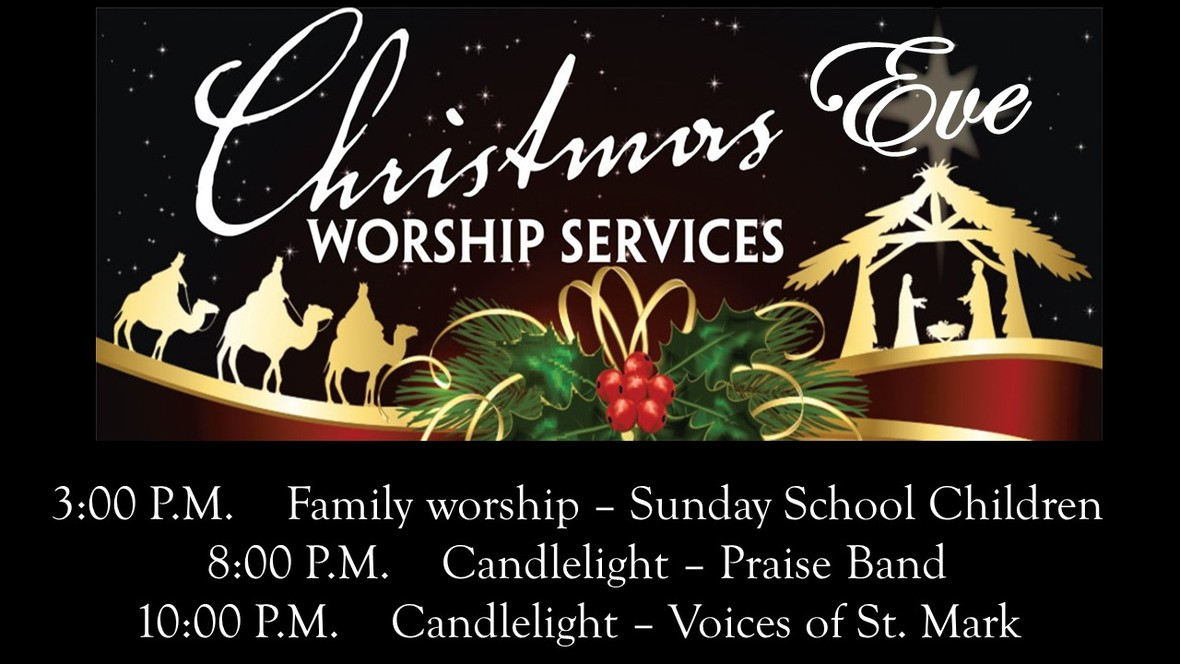Please take note of the Family Worship time change to 3:00 P.M. from 4:30.

 table div table+table+table+table+table+table+table+table+table+table+table+table+table+table div table{width:100%;padding:0}table div table+table+table+table+table+table+table+table+table+table+table+table+table+table div table img{width:96.23%;padding:0;float:none}table div table+table+table+table+table+table+table+table+table+table+table+table+table+table div table td{width:100%;padding:0 1.88% 18px}/* styles *//* styles */ Thank you to the Sunday School children and Karen Kammer for the beautiful Christmas program last Sunday. It is always a special morning to hear and see the children sing.table div table+table+table+table+table+table+table+table+table+table+table+table+table+table+table+table+table div table{width:100%;padding:0}table div table+table+table+table+table+table+table+table+table+table+table+table+table+table+table+table+table div table img{width:96.23%;padding:0;float:none}table div table+table+table+table+table+table+table+table+table+table+table+table+table+table+table+table+table div table td{width:100%;padding:0 1.88% 18px}/* styles */Poinsettias
We are decorating the Sanctuary for the Christmas season with red and white poinsettias. The cost is \$10 per plant. The deadline to order is December 16.

 table div table+table+table+table+table+table+table+table+table+table+table+table+table+table+table+table+table+table+table div table{width:100%;padding:0}table div table+table+table+table+table+table+table+table+table+table+table+table+table+table+table+table+table+table+table div table img{width:96.23%;padding:0;float:none}table div table+table+table+table+table+table+table+table+table+table+table+table+table+table+table+table+table+table+table div table td{width:100%;padding:0 1.88% 18px}/* styles */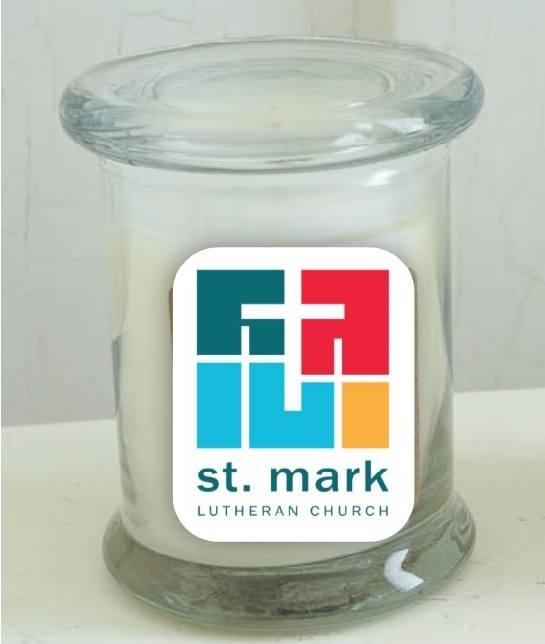Blessing Candles for Christmas!
St. Mark Youth Ministry is selling locally made, soy candles that include our new church logo. Consider a candle for someone that is a blessing in your life, and get one for yourself as a reminder to count your blessings! Candles will be on sale this Sunday, December 16. Cost of each candle is \$16. (we will have a limited number, get them while they last.) Cash or check to St. Mark will be accepted.

 table div table+table+table+table+table+table+table+table+table+table+table+table+table+table+table+table+table+table+table+table+table div table{width:100%;padding:0}table div table+table+table+table+table+table+table+table+table+table+table+table+table+table+table+table+table+table+table+table+table div table img{width:96.23%;padding:0;float:none}table div table+table+table+table+table+table+table+table+table+table+table+table+table+table+table+table+table+table+table+table+table div table td{width:100%;padding:0 1.88% 18px}/* styles */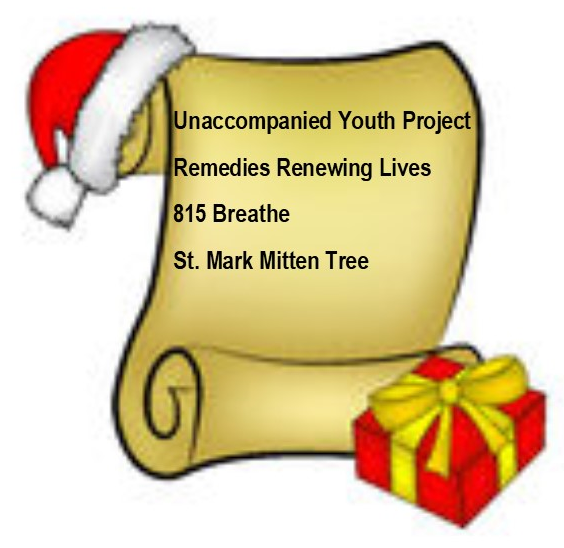St. Mark Christmas Ministry
This week there is still time to turn in the donations for Remedies Women's Shelter(tote bags of toiletries) and 815 Breathe(winter coats for teens).

We will be collecting hats, gloves, scarves, and mittens through the month of December.

Thank you and bless you for the abundance of donations that are coming in. Please place the donations in or around the red truck in Fellowship Hall.

Monetary donations accepted, for all of the above collections. Please make checks to St. Mark and write Christmas Ministry in the memo line. This above will be collected through the month of December.

 table div table+table+table+table+table+table+table+table+table+table+table+table+table+table+table+table+table+table+table+table+table+table+table div table{width:100%;padding:0}table div table+table+table+table+table+table+table+table+table+table+table+table+table+table+table+table+table+table+table+table+table+table+table div table img{width:96.23%;padding:0;float:none}table div table+table+table+table+table+table+table+table+table+table+table+table+table+table+table+table+table+table+table+table+table+table+table div table td{width:100%;padding:0 1.88% 18px}/* styles */Thank you for ALL the wonderful donations for the Unaccompanied Youth Project. The items were delivered to area high school seniors who are homeless. We already heard back from one of the principals that the items were very timely and appreciated.

 table div table+table+table+table+table+table+table+table+table+table+table+table+table+table+table+table+table+table+table+table+table+table+table+table+table div table{width:100%;padding:0}table div table+table+table+table+table+table+table+table+table+table+table+table+table+table+table+table+table+table+table+table+table+table+table+table+table div table img{width:96.23%;padding:0;float:none}table div table+table+table+table+table+table+table+table+table+table+table+table+table+table+table+table+table+table+table+table+table+table+table+table+table div table td{width:100%;padding:0 1.88% 18px}/* styles */Miss a Sunday at St. Mark? Did you know that you can watch the sermons online? Click here to watch.

 table div table+table+table+table+table+table+table+table+table+table+table+table+table+table+table+table+table+table+table+table+table+table+table+table+table+table+table div table{width:100%;padding:0}table div table+table+table+table+table+table+table+table+table+table+table+table+table+table+table+table+table+table+table+table+table+table+table+table+table+table+table div table img{width:96.23%;padding:0;float:none}table div table+table+table+table+table+table+table+table+table+table+table+table+table+table+table+table+table+table+table+table+table+table+table+table+table+table+table div table td{width:100%;padding:0 1.88% 18px}/* styles */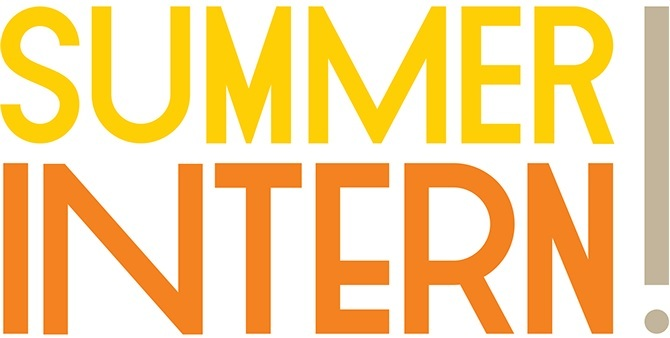Summer Intern
If you're a college-age youth interested in working within the church community, consider applying for St Mark's Youth Summer Intern position. This full-time internship will give one student the opportunity to work in our youth ministries program, Vacation Bible School, and other areas of church life. This unique opportunity includes a stipend funded by the St. Mark Endowment. Anyone interested should contact Pastor Chad. prmckenna@stmarklc.com. Click this link to apply.

 table div table+table+table+table+table+table+table+table+table+table+table+table+table+table+table+table+table+table+table+table+table+table+table+table+table+table+table+table+table div table{width:100%;padding:0}table div table+table+table+table+table+table+table+table+table+table+table+table+table+table+table+table+table+table+table+table+table+table+table+table+table+table+table+table+table div table img{width:96.23%;padding:0;float:none}table div table+table+table+table+table+table+table+table+table+table+table+table+table+table+table+table+table+table+table+table+table+table+table+table+table+table+table+table+table div table td{width:100%;padding:0 1.88% 18px}/* styles */End of the Year Giving
As we near the end of the year, please review your 2018 giving commitment to St. Mark. All gifts must be received in the St. Mark office on or before December 31st to be counted for 2018. And please keep St. Mark in mind in your Christmas giving!
Generous Christmas gifts from our members are a significant part of our yearly giving. Please use your Christmas envelopes! Thank you.

 table div table+table+table+table+table+table+table+table+table+table+table+table+table+table+table+table+table+table+table+table+table+table+table+table+table+table+table+table+table+table+table div table{width:100%;padding:0}table div table+table+table+table+table+table+table+table+table+table+table+table+table+table+table+table+table+table+table+table+table+table+table+table+table+table+table+table+table+table+table div table img{width:96.23%;padding:0;float:none}table div table+table+table+table+table+table+table+table+table+table+table+table+table+table+table+table+table+table+table+table+table+table+table+table+table+table+table+table+table+table+table div table td{width:100%;padding:0 1.88% 18px}/* styles */A reminder that there will be no Wednesday morning men and women's Bible studies on December 26. The church will be closed that day.

 table div table+table+table+table+table+table+table+table+table+table+table+table+table+table+table+table+table+table+table+table+table+table+table+table+table+table+table+table+table+table+table+table+table div table{width:100%;padding:0}table div table+table+table+table+table+table+table+table+table+table+table+table+table+table+table+table+table+table+table+table+table+table+table+table+table+table+table+table+table+table+table+table+table div table img{width:96.23%;padding:0;float:none}table div table+table+table+table+table+table+table+table+table+table+table+table+table+table+table+table+table+table+table+table+table+table+table+table+table+table+table+table+table+table+table+table+table div table td{width:100%;padding:0 1.88% 18px}/* styles */The 2019 altar flower chart is up in Fellowship Hall on the cabinets next to the coffee station on Sunday mornings. The arrangements will be \$35. This is a great way to honor or remember that special someone or that special anniversary.

 table div table+table+table+table+table+table+table+table+table+table+table+table+table+table+table+table+table+table+table+table+table+table+table+table+table+table+table+table+table+table+table+table+table+table+table div table{width:100%;padding:0}table div table+table+table+table+table+table+table+table+table+table+table+table+table+table+table+table+table+table+table+table+table+table+table+table+table+table+table+table+table+table+table+table+table+table+table div table img{width:96.23%;padding:0;float:none}table div table+table+table+table+table+table+table+table+table+table+table+table+table+table+table+table+table+table+table+table+table+table+table+table+table+table+table+table+table+table+table+table+table+table+table div table td{width:100%;padding:0 1.88% 18px}/* styles */Pledge Update: On October 21, we announced our 2019 Congregation Budget goal of \$825,800.00, a small increase of only \$661 over our 2018 budget. To date, we have received 266 pledges, totaling \$727,790.00. This amount represents 97.1% of the \$749,700.00 of pledged giving needed for 2019. Our pledged giving of \$749,700.00 is a key part of our total budget of \$825,800.00. This year’s stewardship campaign will continue each week until our congregation budget goal is reached. We thank you for your participation in this important expression of support and gratitude. Together, we can keep our ministry moving forward with confidence and hope.

If you have not turned in your pledge card for 2019, please do so. With everyone's assistance, we can better anticipate the full level of support from all our members for the coming year.

 table div table+table+table+table+table+table+table+table+table+table+table+table+table+table+table+table+table+table+table+table+table+table+table+table+table+table+table+table+table+table+table+table+table+table+table+table+table div table{width:100%;padding:0}table div table+table+table+table+table+table+table+table+table+table+table+table+table+table+table+table+table+table+table+table+table+table+table+table+table+table+table+table+table+table+table+table+table+table+table+table+table div table img{width:96.23%;padding:0;float:none}table div table+table+table+table+table+table+table+table+table+table+table+table+table+table+table+table+table+table+table+table+table+table+table+table+table+table+table+table+table+table+table+table+table+table+table+table+table div table td{width:100%;padding:0 1.88% 18px}/* styles */If you have a prayer request that you would like the prayer chain to pray for, please send it to Karen Kammer at karen@stmarklc.com. Please be specific about your prayer request. All requests are confidential within the prayer chain. If you have requested prayers from the prayer chain, please notify Karen Kammer of any changes or updates so that our prayers can be most relevant.

 table div table+table+table+table+table+table+table+table+table+table+table+table+table+table+table+table+table+table+table+table+table+table+table+table+table+table+table+table+table+table+table+table+table+table+table+table+table+table+table div table{width:100%;padding:0}table div table+table+table+table+table+table+table+table+table+table+table+table+table+table+table+table+table+table+table+table+table+table+table+table+table+table+table+table+table+table+table+table+table+table+table+table+table+table+table div table img{width:96.23%;padding:0;float:none}table div table+table+table+table+table+table+table+table+table+table+table+table+table+table+table+table+table+table+table+table+table+table+table+table+table+table+table+table+table+table+table+table+table+table+table+table+table+table+table div table td{width:100%;padding:0 1.88% 18px}/* styles */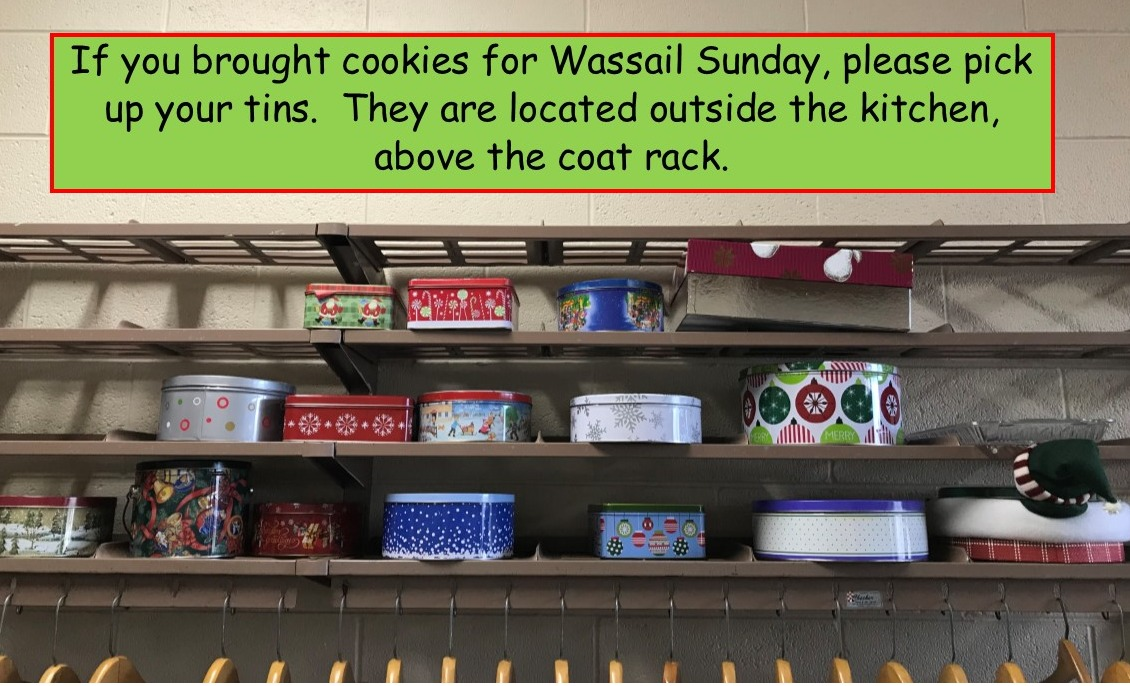table div table+table+table+table+table+table+table+table+table+table+table+table+table+table+table+table+table+table+table+table+table+table+table+table+table+table+table+table+table+table+table+table+table+table+table+table+table+table+table+table+table div table{width:100%;padding:0}table div table+table+table+table+table+table+table+table+table+table+table+table+table+table+table+table+table+table+table+table+table+table+table+table+table+table+table+table+table+table+table+table+table+table+table+table+table+table+table+table+table div table img{width:96.23%;padding:0;float:none}table div table+table+table+table+table+table+table+table+table+table+table+table+table+table+table+table+table+table+table+table+table+table+table+table+table+table+table+table+table+table+table+table+table+table+table+table+table+table+table+table+table div table td{width:100%;padding:0 1.88% 18px}/* styles */## Youth MinistryHigh School Movie Night"
Sunday, December 30, 6-8:30 P.M. at

Second Sunday in the new year will be January 13, 7-8:30 P.M. Join us at Katie's Cup, each Second Sunday of the month. If you need a ride please just message Melinda Alekna at 815-871-0390 and we will pick you up.

Youth Connect is planning a fun event at Urban Air Trampoline and Adventure Park. Sunday, January 27th, 2019! We will be carpooling that day and leaving at 12:30 P.M. Watch for more details. This event is for high/middle school youth. See Melinda Alekna if you have questions.

St. Mark High School Youth High Adventure Trip June 23-28, 2019. We will be traveling to the Apostle Islands, in northern Wisconsin. Watch for more details! This is for young people entering high school fall 2019 and those in high school. There will be an informational meeting on Sunday, January 6, 2019, at 10:45 A.M.

Reserve your spot at a reduced deposit of \$50 by December 25, after this date it will go up to \$100 deposit.

 table div table+table+table+table+table+table+table+table+table+table+table+table+table+table+table+table+table+table+table+table+table+table+table+table+table+table+table+table+table+table+table+table+table+table+table+table+table+table+table+table+table+table+table+table div table{width:100%;padding:0}table div table+table+table+table+table+table+table+table+table+table+table+table+table+table+table+table+table+table+table+table+table+table+table+table+table+table+table+table+table+table+table+table+table+table+table+table+table+table+table+table+table+table+table+table div table img{width:96.23%;padding:0;float:none}table div table+table+table+table+table+table+table+table+table+table+table+table+table+table+table+table+table+table+table+table+table+table+table+table+table+table+table+table+table+table+table+table+table+table+table+table+table+table+table+table+table+table+table+table div table td{width:100%;padding:0 1.88% 18px}/* styles */## Children's MinistryThere is no Sunday school on December 30.

Sunday School Singers will sing Christmas carols during the Prelude and The Virgin Mary Had a Baby Boy during the Offertory on Christmas Eve, December 24 at the 3 P.M. service. Students should arrive at 2:30 P.M. in the lower level to warm-up their voices. Students will sit with their families. Parents will need to save seats in the sanctuary for their children.

The Family Worship service time on Christmas Eve has changed from 4:30 P.M. to 3:00 P.M. Mark your calendars! Christmas Eve worship times are 3, 8 and 10 P.M.

The Good News Bearers will perform His Story on Christmas Eve, December 24 at the 3 P.M. service. Bearers should arrive in the lower level at 2 P.M. to prepare and rehearse.

Karen Kammer’s last day of employment is December 31. Julie Eshleman will begin her role as Director of Christian Education on January 2.

 table div table+table+table+table+table+table+table+table+table+table+table+table+table+table+table+table+table+table+table+table+table+table+table+table+table+table+table+table+table+table+table+table+table+table+table+table+table+table+table+table+table+table+table+table+table+table+table div table{width:100%;padding:0}table div table+table+table+table+table+table+table+table+table+table+table+table+table+table+table+table+table+table+table+table+table+table+table+table+table+table+table+table+table+table+table+table+table+table+table+table+table+table+table+table+table+table+table+table+table+table+table div table img{width:96.23%;padding:0;float:none}table div table+table+table+table+table+table+table+table+table+table+table+table+table+table+table+table+table+table+table+table+table+table+table+table+table+table+table+table+table+table+table+table+table+table+table+table+table+table+table+table+table+table+table+table+table+table+table div table td{width:100%;padding:0 1.88% 18px}/* styles */table div table+table+table+table+table+table+table+table+table+table+table+table+table+table+table+table+table+table+table+table+table+table+table+table+table+table+table+table+table+table+table+table+table+table+table+table+table+table+table+table+table+table+table+table+table+table+table+table+table+table div table{width:100%;padding:0}table div table+table+table+table+table+table+table+table+table+table+table+table+table+table+table+table+table+table+table+table+table+table+table+table+table+table+table+table+table+table+table+table+table+table+table+table+table+table+table+table+table+table+table+table+table+table+table+table+table+table div table img{width:96.23%;padding:0;float:none}table div table+table+table+table+table+table+table+table+table+table+table+table+table+table+table+table+table+table+table+table+table+table+table+table+table+table+table+table+table+table+table+table+table+table+table+table+table+table+table+table+table+table+table+table+table+table+table+table+table+table div table td{width:100%;padding:0 1.88% 18px}/* styles */## Our St. Mark members:

Bob Zeschke, Dorothy Brommerich, Linda Swanson, Pam Cottrill, Keith Haggestad, Naomi Hagen.

## Family and friends of St. Mark members:

Steve Larsen, Bill Durkee, Scott Jones, Ethan Whitney, Carolyn King, Tom Wolfe, Tom Severson, Katie Butera, Kate Nickell, Ashton Henley, Nathan Gyllin.

## Those serving in the military and their families:

Bailey Panjkovich, Xander Gehrke, Jim Tammen, John Nelson, Brandon Ballenger, Taylor Eversole, Dean Barron.

 table div table+table+table+table+table+table+table+table+table+table+table+table+table+table+table+table+table+table+table+table+table+table+table+table+table+table+table+table+table+table+table+table+table+table+table+table+table+table+table+table+table+table+table+table+table+table+table+table+table+table+table+table+table div table{width:100%;padding:0}table div table+table+table+table+table+table+table+table+table+table+table+table+table+table+table+table+table+table+table+table+table+table+table+table+table+table+table+table+table+table+table+table+table+table+table+table+table+table+table+table+table+table+table+table+table+table+table+table+table+table+table+table+table div table img{width:96.23%;padding:0;float:none}table div table+table+table+table+table+table+table+table+table+table+table+table+table+table+table+table+table+table+table+table+table+table+table+table+table+table+table+table+table+table+table+table+table+table+table+table+table+table+table+table+table+table+table+table+table+table+table+table+table+table+table+table+table div table td{width:100%;padding:0 1.88% 18px}/* styles *//* styles */ Office Hours Monday - Friday, 8:00 A.M. - 4:00 P.M. Telephone: 815-398-3557 Publications St. Mark Memos, the weekly e-newsletter, is distributed each Thursday. The deadline for submission is Monday by 4:00 P.M. Please click to send submissions. Sermons Online Miss a Sunday at St. Mark? Please visit our website and watch the sermons online. Please click to watch.
 table div table+table+table+table+table+table+table+table+table+table+table+table+table+table+table+table+table+table+table+table+table+table+table+table+table+table+table+table+table+table+table+table+table+table+table+table+table+table+table+table+table+table+table+table+table+table+table+table+table+table+table+table+table+table+table div table{width:100%;padding:0}table div table+table+table+table+table+table+table+table+table+table+table+table+table+table+table+table+table+table+table+table+table+table+table+table+table+table+table+table+table+table+table+table+table+table+table+table+table+table+table+table+table+table+table+table+table+table+table+table+table+table+table+table+table+table+table div table img{width:96.23%;padding:0;float:none}table div table+table+table+table+table+table+table+table+table+table+table+table+table+table+table+table+table+table+table+table+table+table+table+table+table+table+table+table+table+table+table+table+table+table+table+table+table+table+table+table+table+table+table+table+table+table+table+table+table+table+table+table+table+table+table div table td{width:100%;padding:0 1.88% 18px}/* styles */